## Exam given November 22, 2000

Problem 1. Related Rates (20 points)

Water is pouring into a conical vat at a rate of 300 gallons per minute (see picture). The volume V of water is related to the water level h by the formula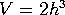. (Here V is measured in gallons and h is measured in feet). At what rate is the water level increasing when the level equals five feet?

Since, we must have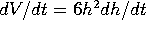, so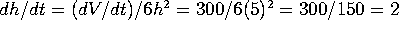feet per minute.

Problem 2. Growth and decay (20 points)

The population of a certain town is growing exponentially. In a certain year (call this t=0), the population is 15,000. Forty years later (t=40), the population is 60,000.

a) By what percent is the town growing each year? In other words, what is the growth rate r (also called k)? Give an exact answer: something like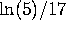, not like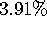.

Since this is exponential growth, we must have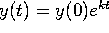. We are told that y(0)=15,000 and that y(40)=60,000. Thus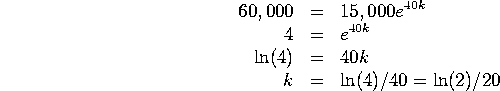Put another way, the population doubles every 20 years.

b) If this exponential growth continues, what will the population of the town be at t=80? Give an exact answer, and then simplify.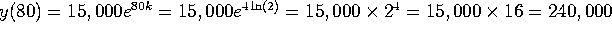. Another way to see this is that the population doubles every 20 years, quadruples every 40 years, and so is multiplied by 16 every 80 years.

[Historical note: the town is Austin, and the starting date is 1880. Austin's population has experienced steady exponential growth for the past 150 years].

Problem 3. Velocity and time (20 points)

A car is moving with velocity dx/dt = 50 + 10 t, where x is measured in miles, t in hours, and dx/dt in miles/hour. At time t=0 the car is at milepost x=100. Where is it at time t=2?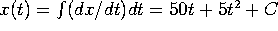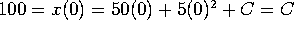, so we must have C=100, so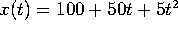, and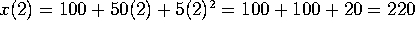.

Problem 4. L'Hopital's rule (20 points)

Evaluate the following three limits:

a)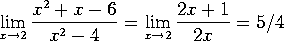.

b)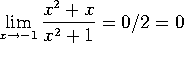. L'Hopital's rule does not apply here.

c)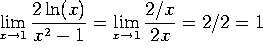.

d)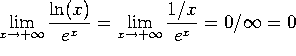Problem 5. Definite integrals (20 points)

We wish to find the area under the curve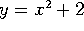between x=0 and x=4.

a) Estimate this area by dividing the interval [0,4] into four pieces and adding the areas of the corresponding rectangles.

Since we are dividing into 4 pieces, we have n=4 and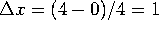. Our points are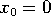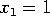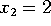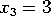and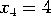. Using upper rectangles, we get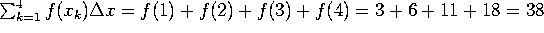. [It is also OK to use lower rectangles, in which case we get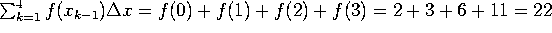.]

b) Estimate the area by dividing the interval into n pieces. Leave your answer as a sum, like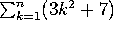. (No, that's not the right answer). You do NOT need to evaluate this sum.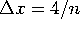, and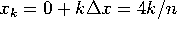. Our sum is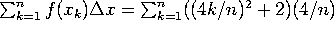. [Alternatively, using lower sums, you could write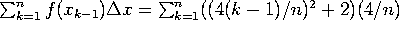].

Problem 6. Indefinite integrals (20 points)

Evaluate the following indefinite integrals

a)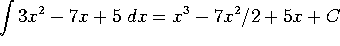b)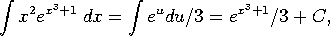where we have used the substitution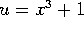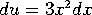.

c)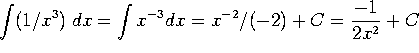.

d)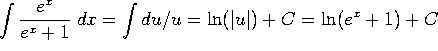,

where we have used the substitution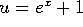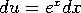.